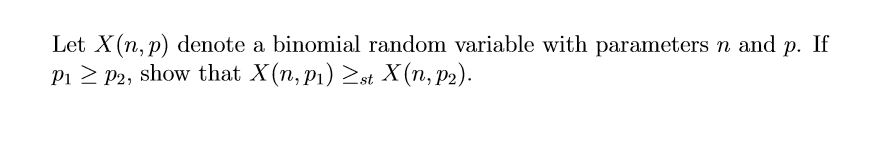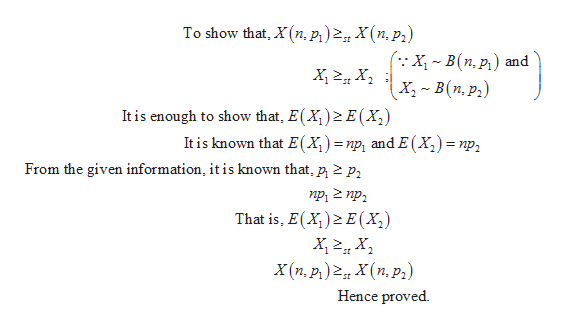# Let X(n, p) denote a binomial random variable with parameters n and p. IfP1 P2, show that X(n, p) st X(n, p2)

Question
177 views

I asked the attached question earlier today, and a response was sent, but none of the answer appeared.  It shows the green check mark as if it has been answered, but nothing followed.help_outlineImage TranscriptioncloseLet X(n, p) denote a binomial random variable with parameters n and p. If P1 P2, show that X(n, p) st X(n, p2) fullscreen
check_circle

Step 1

Introduction to binomial distribution:

Consider a random experiment involving n independent trials, such that the outcome of each trial can be classified as either a “success” or a “failure”. The numerical value “1” is assigned to each success and “0” is assigned to each failure.

Moreover, the probability of getting a success in each trial, p, remains a constant for all the n trials. Denote the probability of failure as q. As success and failure are mutually exclusive, q = 1 – p.

Let the random variable X denote the number of successes obtained from the n trials. Thus, X can take any of the values 0,1,2,…,n.

Then, the probability distribution of X is a Binomial distribution with parameters (n, p) and the probability mass function (pmf) of X, that is, of a Binomial random variable, is given as:

Step 2

Given information:

Here, it is given that X(n,p) is a binomial random variable with parameters n and p.

Consider a binomial random variable X1= X(n,p1).

Consider another binomial random variable X2= X(n,p2).

If X(n,p) is a binomial random variable, then the mean and variance for the binomial variate X are E(x) = n * p and V(x) = n *p *(1 – p).

Since, X1 is a binomial random variable with parameters n and p1, the mean of the binomial random variable X1 is E(X1) = np1.

Since, X2 is a binomial random variable with parameters n and p2, the mean of the binomial random variable X2 is E(X2) = np2.

Step 3

Calculation to show that X(n,p1) ≥st X(n,p2):

From the below given calculation, it is proved that X(n,p1) ≥st X(n,p2):

...help_outlineImage TranscriptioncloseTo show that, X(n, p1)X(n,p) :X- В(п,р.) х, ~ в (п, р,) and It is enough to show that, E(X2 E(X It is known that E(X =np and E (X) = np From the given information, it is known that, p 2 p, duz du That is, E(X) E(X2) X(п, р.)2 X (п,р,) Hence proved fullscreen

### Want to see the full answer?

See Solution

#### Want to see this answer and more?

Solutions are written by subject experts who are available 24/7. Questions are typically answered within 1 hour.*

See Solution
*Response times may vary by subject and question.
Tagged in

### Other